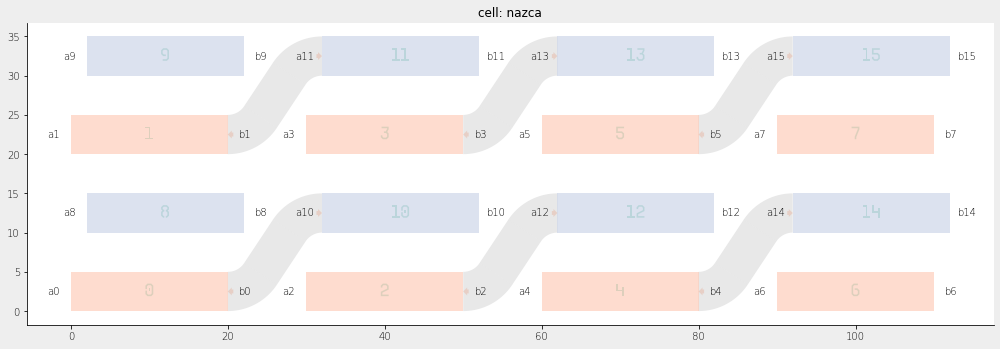Home Forums Nazca Connect polygons corresponding to different layers

Viewing 7 posts - 1 through 7 (of 7 total)
• Author
Posts
• #6477

Dear Nazca team,

I am new to Nazca framework and trying to use it in order to modify an existing *gds file.  It has two layers, each containing equally spaced horizontal polygons along y-axis and shifted along x-axis in one layer with respect to the other. I am struggling with how to connect the beginning of every (let’s say) 4th polygon from the ‘first’ layer with the end of every 4th polygon from the ‘second’ layer without touching other polygons and put the ‘connectors’ in another layer. Any help would be very much appreciated.

#6479

Dear A,

A picture would help, but in general, for using interconnects in an existing gds one has load the gds in a Nazca cell and assign Nazca pins to the cell at positions in the gds that matches the polygon positions to give a meaning to it. This tutorial on creating a building block from gdsmay help.

For implementing xsection in one or more specific gds layer(s), which you can use in interconnects see xsections_and_layers.

There is also a tutorial on interconnectson how to use the interconnects to connect pins in your layout.

For general concepts see getting_started.

Regards,
Ronald

#6480

Dear Ronald,

Thank you very much for your reply on the forum. I am aware of most of the links you provided but I still need a little help. Indeed, a picture would explain better what I am trying to achieve.

Please see below the sketch of the gds design. It has two layers (in red and blue colors), each containing spaced horizontal polygons shifted along x-axis in one layer with respect to the other. I would like to iterate over these polygons in the existing gds file and connect the beginning of every 2th polygon from the ‘red’ layer with the end of every 2th polygon from the blue layer and put the connectors (metal lines) in another layer (yellow color).

``````import nazca as nd
import nazca.geometries as geom
from nazca.interconnects import Interconnect

def rect1(layer_n=1):
rect_shape = nd.geom.rectangle(length=20, height=5)
with nd.Cell('rect1') as cell:
nd.Polygon(layer=1, points=rect_shape).put(0)
nd.Polygon(layer=10, points=rect_shape).put(2, 10)
# guide=ic.sbend_p2p(pin1=r1, pin2=r2.pin['a0']).put()
return cell

rect1().put(array=[4, [30, 0], 2, [0, 20]])

nd.export_plt()``````

I know how to open and change a gds file. The difficulty I have is how to iterate over the polygons from different layers and connect them?

Thank you very much for your help.
A#6481

Hi A,

Thanks for the picture.

Do you know the polygon positions in advance, or would you have to extract them from the gds?

Ronald

#6482

Hi Ronald,

I do not know the polygon positions in advance.

A

#6485

Hi A,

Below is an example to achieve what you want. Depending on your polygons etc. you may want to add a more fancy polygon analysis, but that is not Nazca specific.

``````import nazca as nd
import nazca.geometries as geom
from nazca.interconnects import Interconnect

def rect1(layer_n=1):
rect_shape = nd.geom.rectangle(length=20, height=5)
with nd.Cell('rect1') as cell:
nd.Polygon(layer=1, points=rect_shape).put(0)
nd.Polygon(layer=10, points=rect_shape).put(2, 10)
# guide=ic.sbend_p2p(pin1=r1, pin2=r2.pin['a0']).put()
return cell

rect1().put(array=[4, [30, 0], 2, [0, 20]])  # This could be a gds_load() based cell.

# 1. Extract all the polygon from cell into a dict with key (layer, x, y),
#    where x and y will be, just a choice, the geometrical average of the polygon points.
polygons = {}
for params in nd.cell_iter(nd.cfg.topcell, flat=True):
for pgon, points, bbox in params.iters['polygon']:
xp, yp = zip(*points)
xavg, yavg = round(sum(xp) / len(xp), 2), round(sum(yp) / len(yp), 2)
polygons[(pgon.layer, xavg, yavg)] = points

# 2. Analyse polygons: order them and extract positions for pins.
for i, ((layer, x, y), points) in enumerate(sorted(polygons.items())):
nd.text(str(i), height=2, align='cc', layer=0).put(x, y)
sort = sorted(points)
x0 = sort + 0.5 * (sort - sort)
y0 = sort + 0.5 * (sort - sort)
w0 = abs(sort - sort)
nd.Pin(f'a{i}', width=w0).put(x0, y0, 180)
x1 = sort[-1] + 0.5 * (sort[-2] - sort[-1])
y1 = sort[-1] + 0.5 * (sort[-2] - sort[-1])
nd.Pin(f'b{i}', width=w0).put(x1, y1, 0)

for i, (name, pin) in enumerate(nd.cfg.topcell.pin.items()):
if i in [0, 1, 2, 3, 4, 5]:
ic.bend_strt_bend_p2p(
pin1=nd.cfg.topcell.pin[f'b{i}'],
pin2=nd.cfg.topcell.pin[f'a{i+10}'],
).put()

nd.export_plt()``````

Note that it would make sense to put item 2 and 3 in the script above inside a new cell `with nd.Cell("newcellname") as C:`, and work with cell C, rather than working on the default topcell under nd.cfg.topcell.

Ronald#6501

Hi Ronald,